# Bounded colorings for planar graphs (Solved)

 Importance: Medium ✭✭
 Author(s): Alon, Noga Ding, Guoli Oporowski, Bogdan Vertigan, Dirk
 Subject: Graph Theory » Topological Graph Theory » » Coloring
 Keywords: coloring partition planar graph
 Posted by: mdevos on: May 23rd, 2007
 Solved by: Louis Esperet, Gwenaël Joret [arXiv:1303.2487]
Question   Does there exists a fixed functionso that every planar graph of maximum degreehas a partition of its vertex set into at most three setsso that for, every component of the graph induced by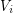has size at most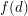?

An ordinary-coloring of a graph, is a partition the vertex set into at mostsets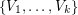so that eachinduces a subgraph where every component is an isolated vertex. Here we are considering a relaxation of this where we insist only that eachinduces a subgraph where every component has bounded size. This type of coloring was first suggested by Ding, Oporowski, and Vertigan and has already lead to some interesting results see [ADOV] and [HSzT].

It follows from the four color theorem that every planar graph has a vertex partition into four setsso that every component of a subgraph induced by somehas size at most 1. On the other hand, Alon, Ding, Oporowski, and Vertigan construct for every integera planar graphwith the property that for every partition of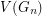into, some component of a subgraph induced by somewill have size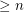. The construction is easy to give: Letbe the graph obtained from a pathof length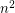by adding a new vertexadjacent to every vertex of this path. Now, form the graphfromdisjoint copies ofby adding a new vertexjoined to every old vertex. Note thatis planar as required. Let us assume (for a contradiction) thatis a partition ofwith the property that every component of a subgraph induced byhas size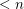. We may assume without loss that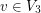, but then there are at most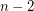other vertices in, so there is a copy ofwhich contains no vertices in. We may assume that the vertexin this copy is in the set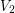, but then at mostother vertices in the corresponding copy ofare in. It now follows that there is a subpath of this copy ofof lengthin which all vertices are in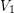. This completes the proof. The above question asks if such a family can still be constructed with the additional constraint that all vertices have bounded degree.

In 2013, this question was answered in the affirmative by Louis Esperet and Gwenaël Joret [EJ].

## Bibliography

*[ADOV] Alon, Noga; Ding, Guoli; Oporowski, Bogdan; Vertigan, Dirk, Partitioning into graphs with only small components, J. Combin. Theory Ser. B 87 (2003), no. 2, 231--243. MathSciNet

[HSzT] Haxell, Penny; Szabó, Tibor; Tardos, Gábor, Bounded size components - partitions and transversals. J. Combin. Theory Ser. B 88 (2003), no. 2, 281--297. MathSciNet

[EJ] Louis Esperet, Gwenaël Joret. Coloring planar graphs with three colors and no large monochromatic components, arXiv:1303.2487, 2013.

* indicates original appearance(s) of problem.